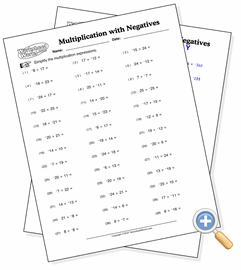# Multiplication With NegativesMaster basic math operations with negative numbers

This exercise helps students get started understanding that negative numbers can be multiplied with one another. They will first need to understand that the result of multiplying a negative with a positive is always negative and that the result of multiplying two negatives is always positive. Depending on your settings below, some regular (non-negative) multiplication problems will be added to the page.